### Simulation

2019.09.11

・SPICE models include thermal models which are used to perform simulations relating to heat.

・A thermal model is a model of an electrical circuit that corresponds to transient thermal resistances, for use in calculations of the thermal circuit associated with the electrical circuit.

・By applying the power dissipation Pd as a current I to a thermal model Rth, the junction temperature Tj can be monitored as corresponding to a voltage.

Among SPICE models, there are what are called thermal models and thermal dynamic models, which are used to perform simulations relating to heat. We first describe thermal models. We hope the reader will acquire a conceptual image of the general nature of thermal models.

## What is Thermal Model?

A thermal model is a model of an electrical circuit that corresponds to transient thermal resistances, for use in calculations of the thermal circuit associated with the electrical circuit. Thermal resistances are represented by R, and thermal capacities by C. The interchange of thermal circuits and electrical circuits is as follows.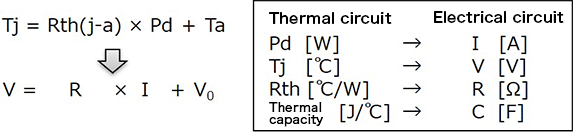From the equations, by applying the power dissipation as a current I to a thermal model Rth, the junction temperature Tj can be monitored as corresponding to a voltage.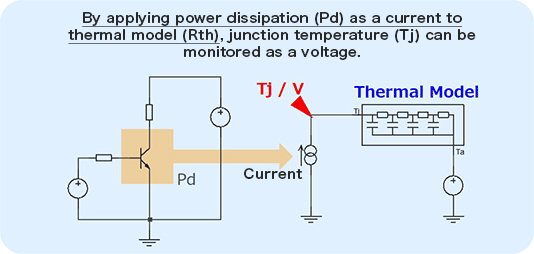For reference, we present thermal models for both surface-mount type and through-hole-type circuits.
Surface-mount type models are Rth(j-a) models for simulations from the chip to the board, and through-hole-type models are Rth(j-c) for simulations from the chip to the frame.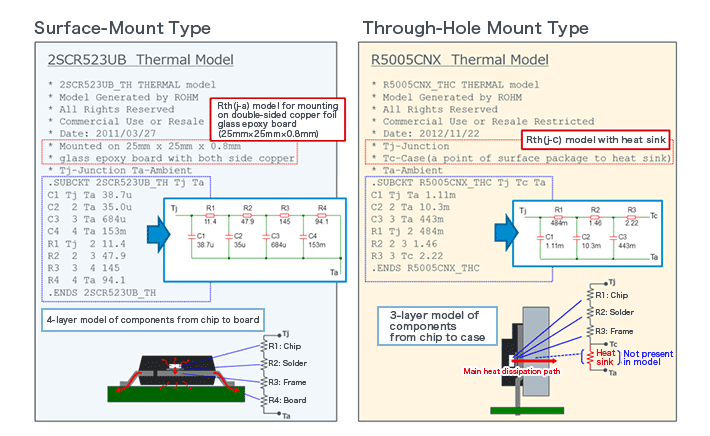There are cases in which a thermal model is included in the datasheet for a device. Upon clicking on the datasheet on the right, an enlarged view appears, and you will see a table indicated by an arrow and a circuit diagram below the table.

The graph below shows a simulation of the characteristics of a thermal model (transient thermal resistance) of the 2SCR523UB (an NPN transistor for general amplification uses) described earlier. A constant 1 A current is applied in steps, and the voltage at pin Tj is monitored.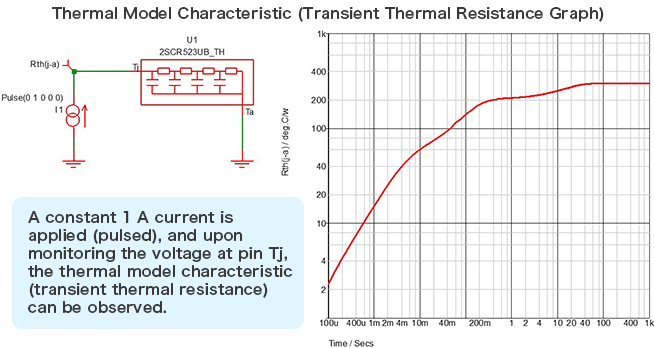Next, we describe an example of use of the thermal model. In this example, the power Pd and junction temperature Tj of the transistor are monitored. As Ta, the relevant bias voltage, in this case 25°C = 25 VDC, is applied.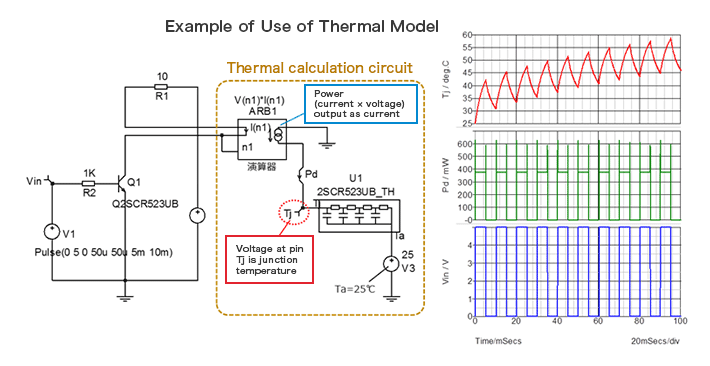As the uppermost graph indicates, the temperature of the switching transistor 2SCR523UB rises while being linked with the switching. In this way, transient changes in Tj can be monitored.